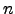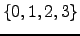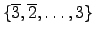Homework IV

Tom Kelliher, CS 240

50 points, due Mar. 12

1. (10 pts.) 3-40. Use only the logic building blocks mentioned in the problem.

2. (10 pts.) 4-11. Hint: This is a circuit with eight inputs. You probably dont't want to construct the truth table for this circuit. Instead, consider a modular divide-and-conquer approach, similar to how one uses divide-and-conquer when designing an-bit adder fromone-bit full adders. Your one-bit module will have three single-bit inputs and one single-bit output; you'll have to decide what the inputs and output represent. You will need to carefully consider whether the module outputs propagate from the least significant bit toward the most significant bit (a la addition) or in the opposite direction.

3. (20 pts.) Design three 64-bit adders, including figures showing the connections between components:

How would the delays for your three adders generalize to an-bit adder?
4. (10 pts.) The radix four digit set is, of course,. The maximally redundant signed digit radix four digit set is. Show that it is possible to design an adder for this digit set, such that a carry propagates at most one digit position, by designing the addition table for the sum of two maximally redundant signed digit radix four digits. Hint: This is far easier than the radix two case.
Tom Kelliher